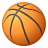# Getting Wrong Result using Spatial Filter in SQL Query

2896
1
Jump to solution
08-22-2015 11:41 PMOccasional Contributor

Hi,

I have a python script where I am executing SELECT SQL statement, using Spatial filter.

The geometry I am using in Spatial filter is being passed as parameter to the python Script.

Now the Issue IS::

Query is running fine. But:

1. for some of the Geometries,SQL is not giving correct output, as compared to the same SpatialFilter applied in ArcMap. E.g. SQL Return 3 where as ArcMap Returns 4. and I can clearly visualize in ArcMap, that feature is overlaping.
2. So to overcome above issue, I commented out the Code of SimplifyPolygon in my Python, and I used original Geom. Now the Result is coming Correct, same as What I am getting in ArcMap.
3. Now this leads to another issue.:: For some of the geometries. It gives me below error while executing SQL Query:
 `ArcSDESQLExecute: StreamPrepareSQL ArcSDE Extended error 1704 ORA-01704: string literal too long`

`scratch = arcpy.env.scratchGDBpolyLayer = os.path.join(scratch,"inputPoly")simplePolyLayer = os.path.join(scratch,"simplePoly")arcpy.MakeFeatureLayer_management(parameters.valueAsText, polyLayer)arcpy.cartography.SimplifyPolygon(polyLayer, simplePolyLayer,"POINT_REMOVE", 5)count = int(arcpy.GetCount_management(simplePolyLayer).getOutput(0))arcpy.AddMessage("count: " + str(count))if count > 1:     #need to merge into a multipolygon then get the WKT     merged = os.path.join(scratch,"mergedPoly")     arcpy.Dissolve_management(simplePolyLayer, merged)     for row in arcpy.da.SearchCursor(merged, ["SHAPE@WKT"]):          WKT = rowelse:     for row in arcpy.da.SearchCursor(simplePolyLayer, ["SHAPE@WKT"]):          WKT = row`

SQL Query code.

`"SELECT count(*) FROM " +<My_FeatureClass> +" a WHERE served_id = 0 and (sdo_anyinteract(a.shape,sdo_geometry('" + WKT + "',4326)) = 'TRUE')"`

Let me know any solutions.

Thanks

Message was edited by: Ravindra Singh

Tags (4)
1 Solution

Accepted SolutionsOccasional Contributor

Hi,

I found the solution regarding this.

Following are the steps you need to do.

1. Download most Suitable version as per your system  of cx_Oracle
2. Install the above . It will be installed at default location-   "C:\Python27\ArcGIS10.2\Lib\site-packages" .  Let it install at here only.
3. NOTE::    If any ESRI product is already opened. Then close it, then Open ArcMap, to let the changes take effect.
4. now modify your script as below:

# Import the package as below

import arcpy, os, sys, arcpy.cartography

#Importing package for making SQL Connection with Oracle

import cx_Oracle

# -- [Strart] Code to get the Geometry as WKT .

scratch = arcpy.env.scratchGDB

polyLayer = os.path.join(scratch,"inputPoly")

arcpy.MakeFeatureLayer_management(parameters.valueAsText, polyLayer)

count = int(arcpy.GetCount_management(polyLayer).getOutput(0))

arcpy.AddMessage("count: " + str(count))

if count > 1:

#need to merge into a multipolygon then get the WKT

merged = os.path.join(scratch,"mergedPoly")

arcpy.Dissolve_management(polyLayer, merged)

for row in arcpy.da.SearchCursor(merged, ["SHAPE@WKT"]):

WKT = row

else:

for row in arcpy.da.SearchCursor(polyLayer, ["SHAPE@WKT"]):

WKT = row

# -- [END] Code to get the Geometry as WKT .

# Create Oracle Connection.

connection = cx_Oracle.connect('<Database_UserID>/<pwd>@<Service_Name>')

cursor = connection.cursor()

mySpatialSQLQuery = "SELECT count(*) FROM PLOTS a WHERE served_id = 0 and (sdo_anyinteract(a.shape,sdo_geometry(:WKTGeom,4326)) = 'TRUE')"

#in the above query ':WKYGeom' is the place holder whose value we will pass in next.

WKTGeom = WKT

cursor.setinputsizes(WKTGeom=cx_Oracle.CLOB)              # prepare memory for operation parameters

cursor.execute(plotCountSQL, {'WKTGeom':WKTGeom})   # Executing using Binding Variable using CLOB

res = cursor.fetchall()

plotCount = res               # It Denotes First Row's First Column

#arcpy.AddMessage("plotCount using cx_Oracle== "+ str(plotCount))

cursor.close()

Like this you can pass the huge geometry as WKT format.

Hope this will help someone.Cheers !

1 ReplyOccasional Contributor

Hi,

I found the solution regarding this.

Following are the steps you need to do.

1. Download most Suitable version as per your system  of cx_Oracle
2. Install the above . It will be installed at default location-   "C:\Python27\ArcGIS10.2\Lib\site-packages" .  Let it install at here only.
3. NOTE::    If any ESRI product is already opened. Then close it, then Open ArcMap, to let the changes take effect.
4. now modify your script as below:

# Import the package as below

import arcpy, os, sys, arcpy.cartography

#Importing package for making SQL Connection with Oracle

import cx_Oracle

# -- [Strart] Code to get the Geometry as WKT .

scratch = arcpy.env.scratchGDB

polyLayer = os.path.join(scratch,"inputPoly")

arcpy.MakeFeatureLayer_management(parameters.valueAsText, polyLayer)

count = int(arcpy.GetCount_management(polyLayer).getOutput(0))

arcpy.AddMessage("count: " + str(count))

if count > 1:

#need to merge into a multipolygon then get the WKT

merged = os.path.join(scratch,"mergedPoly")

arcpy.Dissolve_management(polyLayer, merged)

for row in arcpy.da.SearchCursor(merged, ["SHAPE@WKT"]):

WKT = row

else:

for row in arcpy.da.SearchCursor(polyLayer, ["SHAPE@WKT"]):

WKT = row

# -- [END] Code to get the Geometry as WKT .

# Create Oracle Connection.

connection = cx_Oracle.connect('<Database_UserID>/<pwd>@<Service_Name>')

cursor = connection.cursor()

mySpatialSQLQuery = "SELECT count(*) FROM PLOTS a WHERE served_id = 0 and (sdo_anyinteract(a.shape,sdo_geometry(:WKTGeom,4326)) = 'TRUE')"

#in the above query ':WKYGeom' is the place holder whose value we will pass in next.

WKTGeom = WKT

cursor.setinputsizes(WKTGeom=cx_Oracle.CLOB)              # prepare memory for operation parameters

cursor.execute(plotCountSQL, {'WKTGeom':WKTGeom})   # Executing using Binding Variable using CLOB

res = cursor.fetchall()

plotCount = res               # It Denotes First Row's First Column

#arcpy.AddMessage("plotCount using cx_Oracle== "+ str(plotCount))

cursor.close()

Like this you can pass the huge geometry as WKT format.

Hope this will help someone.Cheers !